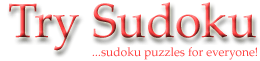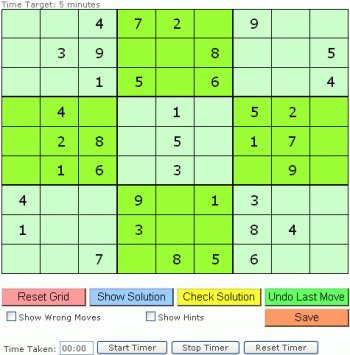# Sudoku Strategy

 The easiest way to solve a sudoku puzzle is by elimination. You know that each row, column and 3 x 3 square contains the numbers one to nine once only. Therefore use the technique called CROSS-HATCHING. This is where you cross-reference each number in a row, column or grid, and try and deduce where each number must go by noting down the options. For instance, in the grid below you can work out that the first number in the centre mini-grid (the top left number of the middle white square) is the number 2. How? Well, in the 3 x 3 square, the numbers 1,3,4,6,9 are present. This leaves the options of 2,5,7,8 for this top left square. However, in the row, the numbers 5 and 8 are also present, leaving 2 and 7. However, in the column, the number 7 is present. This only leaves 2 left, so this must be the value of that square. Using this technique, and checking the possible value of every individual cell is all that you need, combined with patience, to solve many sudoku puzzles.